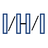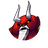Welcome to our new forum
All users of the legacy CODESYS Forums, please create a new account at account.codesys.com. But make sure to use the same E-Mail address as in the old Forum. Then your posts will be matched.

Convert string to byte array. document.SUBSCRIPTION_OPTIONS = { "thing": "topic", "subscribed": false, "url": "subscribe", "icon": { "css": "fa fa-envelope-o" } };

damian177
2021-12-26
2021-12-29
•damian177 - 2021-12-26

Hi,
'AFDA0909090909'
to byte array:
0xAF, 0xDA, 0x09, 0x09, 0x09, 0x09, 0x09

?

•damian177 - 2021-12-26

My temporary solution:

FUNCTION ASCIIstring_TO_BYTE : BYTE
VAR_INPUT
InputVal1: STRING(1);
InputVal2: STRING(1);
END_VAR
VAR
CharAsByteH:BYTE;
CharAsByteL:BYTE;
state1:BYTE;
state2:BYTE;

END_VAR

state1 := 0;
state2 := 0;

IF InputVal1 = '0' THEN   state1 := 1; END_IF;
IF InputVal1 = '1' THEN   state1 := 2; END_IF;
IF InputVal1 = '2' THEN   state1 := 3; END_IF;
IF InputVal1 = '3' THEN   state1 := 4; END_IF;
IF InputVal1 = '4' THEN   state1 := 5; END_IF;
IF InputVal1 = '5' THEN   state1 := 6; END_IF;
IF InputVal1 = '6' THEN   state1 := 7; END_IF;
IF InputVal1 = '7' THEN   state1 := 8; END_IF;
IF InputVal1 = '8' THEN   state1 := 9; END_IF;
IF InputVal1 = '9' THEN   state1 := 10; END_IF;
IF InputVal1 = 'A' THEN   state1 := 11; END_IF;
IF InputVal1 = 'B' THEN   state1 := 12; END_IF;
IF InputVal1 = 'C' THEN   state1 := 13; END_IF;
IF InputVal1 = 'D' THEN   state1 := 14; END_IF;
IF InputVal1 = 'E' THEN   state1 := 15; END_IF;
IF InputVal1 = 'F' THEN   state1 := 16; END_IF;

IF InputVal2 = '0' THEN   state2 := 1; END_IF;
IF InputVal2 = '1' THEN   state2 := 2; END_IF;
IF InputVal2 = '2' THEN   state2 := 3; END_IF;
IF InputVal2 = '3' THEN   state2 := 4; END_IF;
IF InputVal2 = '4' THEN   state2 := 5; END_IF;
IF InputVal2 = '5' THEN   state2 := 6; END_IF;
IF InputVal2 = '6' THEN   state2 := 7; END_IF;
IF InputVal2 = '7' THEN   state2 := 8; END_IF;
IF InputVal2 = '8' THEN   state2 := 9; END_IF;
IF InputVal2 = '9' THEN   state2 := 10; END_IF;
IF InputVal2 = 'A' THEN   state2 := 11; END_IF;
IF InputVal2 = 'B' THEN   state2 := 12; END_IF;
IF InputVal2 = 'C' THEN   state2 := 13; END_IF;
IF InputVal2 = 'D' THEN   state2 := 14; END_IF;
IF InputVal2 = 'E' THEN   state2 := 15; END_IF;
IF InputVal2 = 'F' THEN   state2 := 16; END_IF;

CASE state1 OF
1 : CharAsByteH:=16#00;
2 : CharAsByteH:=16#01;
3 : CharAsByteH:=16#02;
4 : CharAsByteH:=16#03;
5 : CharAsByteH:=16#04;
6 : CharAsByteH:=16#05;
7 : CharAsByteH:=16#06;
8 : CharAsByteH:=16#07;
9 : CharAsByteH:=16#08;
10 : CharAsByteH:=16#09;
11 : CharAsByteH:=16#0A;
12 : CharAsByteH:=16#0B;
13 : CharAsByteH:=16#0C;
14 : CharAsByteH:=16#0D;
15 : CharAsByteH:=16#0E;
16 : CharAsByteH:=16#0F;
END_CASE;

CASE state2 OF
1 : CharAsByteL:=16#00;
2 : CharAsByteL:=16#01;
3 : CharAsByteL:=16#02;
4 : CharAsByteL:=16#03;
5 : CharAsByteL:=16#04;
6 : CharAsByteL:=16#05;
7 : CharAsByteL:=16#06;
8 : CharAsByteL:=16#07;
9 : CharAsByteL:=16#08;
10 : CharAsByteL:=16#09;
11 : CharAsByteL:=16#0A;
12 : CharAsByteL:=16#0B;
13 : CharAsByteL:=16#0C;
14 : CharAsByteL:=16#0D;
15 : CharAsByteL:=16#0E;
16 : CharAsByteL:=16#0F;
END_CASE;

ASCIIstring_TO_BYTE:= SHL(CharAsByteH,4) OR CharAsByteL;

And function call:

byteMy:=ASCIIstring_TO_BYTE('A','F');

I need more profesional solution. :)

Last edit: damian177 2021-12-26
•h-hermsen - 2021-12-26

It seems your solution is KISS, it is readable, understandable and it works. Problem solved if you ask me 💥✨🌟👍💪 Happy Christmas ⛄🎄

👍
1
•h-hermsen - 2021-12-29

I just knew people weren't going to let this go by :-)
The for loop solution js offcourse more elegant and shorter. Anyway Happy New Year!

•dFx - 2021-12-27

Did you had a look at OSCATT lib ? HEX_TO_BYTE seems to apply.

Also there is WORD_AS_STRING in utils, but you need the exact opposite.

Last edit: dFx 2021-12-27
•damian177 - 2021-12-27

... This examle works:

byteMy:=ASCIIstring_TO_BYTE('A','F');

But in this example is problem:

VAR
ASCIIarray:STRING:='DFAFFF0001';
bytes_array: ARRAY[0..100] OF BYTE;
END_VAR

FOR iCount:=0 TO 10 DO
IF (iCount MOD 2) = 0 THEN
bytes_array[iCountTT] := ASCIIstring_TO_BYTE((ASCIIarray[iCount]),(ASCIIarray[iCount+1]));
iCountTT:=iCountTT+1;
END_IF
END_FOR

The error is:
Cannot convert type 'BYTE' to type 'STRING(1)' ...

Function STRING_TO_BYTE not resolved problem. How do I pass one character from STRING to a function?

Last edit: damian177 2021-12-27
•dFx - 2021-12-27

actually, looping from 0 to 10 is 11 loops on a string of 10 chars.

then if you want to get a byte value, don't concatenate 2 chars into a word. just take lower byte once at a time.

•damian177 - 2021-12-27

So how do I pass a single character from string to my function named: ASCIIstring_TO_BYTE?

•damian177 - 2021-12-27

So, maybe have you idea how convert this 1D Array :

ASCIIarray : STRING(255) := 'AFDFAFFFFF';

to 2D ARRAY:

ASCIIarray2 : ARRAY[0..0, 0..4] OF STRING := ['AF', 'DF', 'AF', 'FF', 'FF'];

?

•dFx - 2021-12-29
FOR Index := 0 TO 9 DO
MyBytes[Index] := HEXinASCII_TO_BYTE(MyStr[Index]);
END_FOR

OR

FOR Index := 0 TO 4 DO
MyBytes[Index] := SHL(HEXinASCII_TO_BYTE(MyStr[Index*2]),4) + HEXinASCII_TO_BYTE(MyStr[Index*2 + 1]);
END_FOR

HEXinASCII_TO_BYTE is part of Util lib.

Sometimes things are easier than it first look.

👍
2

Last edit: dFx 2021-12-29
•damian177 - 2021-12-29

Thanks, but what do you think about other conversion. I would like to change byte array :0x44, 0x44, 0x46, 0xFF, 0xFF
on string value :
'444446FFFF' ? How do this ?

•dFx - 2021-12-29
FOR Index := 0 TO 7 DO
MyBytes[Index] := SHL(HEXinASCII_TO_BYTE(MyStr[Index*2]),4) + HEXinASCII_TO_BYTE(MyStr[Index*2 + 1]);
END_FOR
FOR Index := 0 TO 7 DO
MyStr2[Index*2+1] := TO_BYTE(BYTE_TO_HEXinASCII(MyBytes[Index]));
MyStr2[Index*2] := TO_BYTE(BYTE_TO_HEXinASCII(SHR(MyBytes[Index],4)));
END_FOR

•